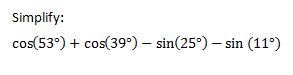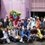Trigonometric IdentitiesHello, I have face a deadlock while trying to find the way to evaluate this identities. I'm curious that we need to use the trigonometry sum formula to find the answer of this problem, but I still cannot find the way to finish it, I always go back to the initial problem. Pls help me, so I can answer my curiosity.. ThanksNote by Leonardo Chandra
6 years, 4 months ago

This discussion board is a place to discuss our Daily Challenges and the math and science related to those challenges. Explanations are more than just a solution — they should explain the steps and thinking strategies that you used to obtain the solution. Comments should further the discussion of math and science.

When posting on Brilliant:

• Use the emojis to react to an explanation, whether you're congratulating a job well done , or just really confused .
• Ask specific questions about the challenge or the steps in somebody's explanation. Well-posed questions can add a lot to the discussion, but posting "I don't understand!" doesn't help anyone.
• Try to contribute something new to the discussion, whether it is an extension, generalization or other idea related to the challenge.
• Stay on topic — we're all here to learn more about math and science, not to hear about your favorite get-rich-quick scheme or current world events.

MarkdownAppears as
*italics* or _italics_ italics
**bold** or __bold__ bold

- bulleted
- list

• bulleted
• list

1. numbered
2. list

1. numbered
2. list
Note: you must add a full line of space before and after lists for them to show up correctly
paragraph 1

paragraph 2

paragraph 1

paragraph 2

> This is a quote
This is a quote
# I indented these lines
# 4 spaces, and now they show
# up as a code block.

print "hello world"
# I indented these lines
# 4 spaces, and now they show
# up as a code block.

print "hello world"
MathAppears as
Remember to wrap math in $$...$$ or $...$ to ensure proper formatting.
2 \times 3 $2 \times 3$
2^{34} $2^{34}$
a_{i-1} $a_{i-1}$
\frac{2}{3} $\frac{2}{3}$
\sqrt{2} $\sqrt{2}$
\sum_{i=1}^3 $\sum_{i=1}^3$
\sin \theta $\sin \theta$
\boxed{123} $\boxed{123}$

Sort by:

You can simplify this to $4sin 13^\circ cos 31^\circ cos 7^\circ$.

You use the following facts to simplify your expression:

$1)$ $sin A + sin B=2sin(\frac{A+B}{2}) cos(\frac{A-B}{2})$

$(2)$ $sin A - sin B=2sin(\frac{A-B}{2}) cos(\frac{A+B}{2})$

$(3)$ $cos A + cos B=2cos(\frac{A+B}{2}) cos(\frac{A-B}{2})$

$(4)$ $cos A - cos B=-2sin(\frac{A+B}{2}) sin(\frac{A-B}{2})$

If you want to know the proofs for these, they are pretty straightforward.

For example:

We can derive, $sin(x+y)+sin(x-y)=2sinxcosy$

Now, let $x+y=A$ and $x-y=B$, and substitute in the above equation.

This will give you the proof for equation $(1)$

- 6 years, 4 months ago

thanks Aditya, now I've just got some knowledge about simplifying the trigonometric identities..:)

- 6 years, 4 months ago

Hint: $53 - 39 = 14 = 25 - 11$, so that suggests a place to start. Likewise, $53 - 25 = 28 = 39 - 11$.

Staff - 6 years, 4 months ago

what is this sir...I cant understand!

- 6 years, 4 months ago

Think about how to apply the hint, esp in light of the sum and product trigonometric formulas that Aditya mentioned.

Since $53 - 39 = 14$, when we combine $\cos 53 + \cos 39$, we will get $\cos \frac{53-39}{2}$ as one of the terms in the product. Since $25 - 11 = 14$, when we combine $- ( sin 25 + \sin 11)$, we will get $\cos \frac{25-11}{2}$ as one of the terms in the product. This allows us to factor out the $\cos 7$ (which you can see in Aditya's answer) and then continue with the remaining terms.

Staff - 6 years, 4 months ago

Aditya your 4th identity is wrong as it should be B-A instead of A-B which is very important as it matters a lot in sin.

- 6 years, 4 months ago

The 4th identity can, alternatively, also have a negative sign, which I supposedly missed while posting my comment. Thanks.

- 6 years, 4 months ago

awh!!

- 4 years, 10 months ago

- 6 years, 4 months ago

don't use a calc...

- 6 years, 4 months ago

Hello..?? We are now in the 21st century... Use calculator or even more sophisticated computing devices like computers installed with math soft wares like mathlab, scilab and python. There are a lot of builtin functions in that three softwares,... one of which you can convert decimal to fraction with higher precision.. you type that in the console, let the software computes...

- 6 years, 4 months ago

You are missing the point. Calculators will only get you an approximate decimal answer. He wants an exact answer in trigonometric form. See Adiya's solution.

Staff - 6 years, 4 months ago